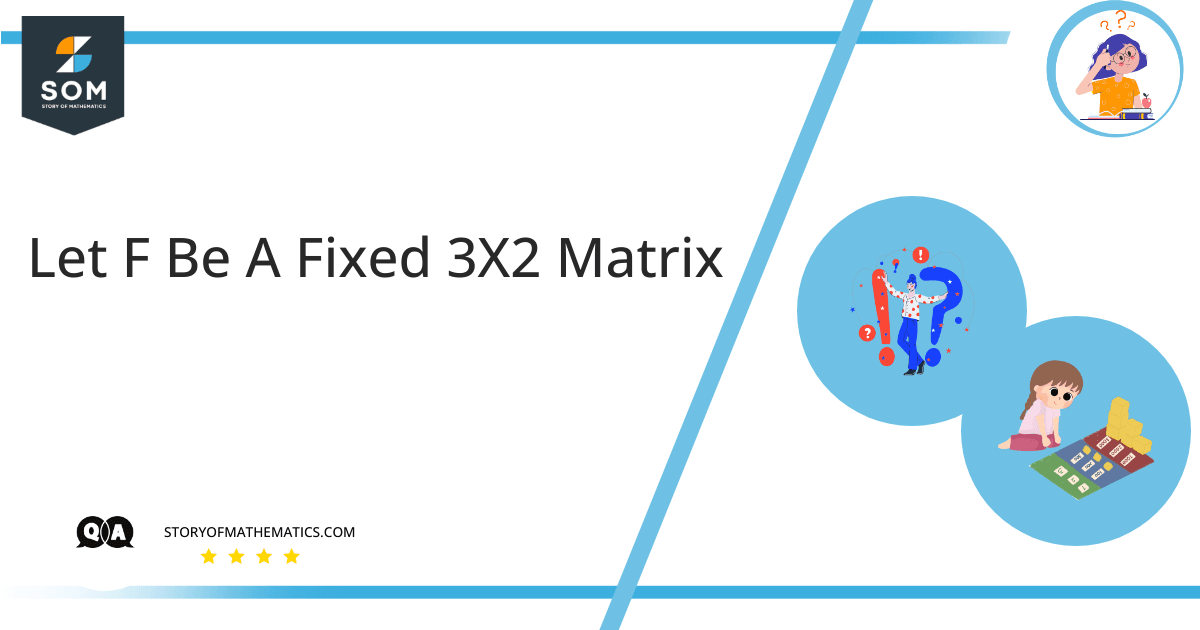# Let f be a fixed 3×2 matrix, and H be the set of matrices A belonging to a 2×4 matrix. If we assume that the property FA = O holds true, show that H is a subspace of M2×4. Here O represents a zero matrix of order 3×4.The aim of this question is to comprehend the key linear algebra concepts of vector spaces and vector subspaces.

vector space is defined as a set of all vectors that fulfill the associative and commutative properties for vector addition and scalar multiplication operations. The minimum no. of unique vectors required to describe a certain vector space is called basis vectors. A vector space is an n-dimensional space defined by linear combinations of basis vectors.

Mathematically, a vector space V must fulfill the following properties:

– Commutative Property of Vector Addition: $u \ + \ v \ = \ v \ + \ u$ where $u$, $v$ are the vectors in $V$

– Associative Property of Vector Addition: $( \ u \ + \ v \ ) \ + \ w \ = \ u \ + \ ( \ v \ + \ w \ )$ where $u$, $v$, $w$ are the vectors in $V$

– Additive Identity: $u \ + \ 0 \ = \ 0 \ + \ u \ = \ u$ where $0$ is the additive identity of $V$

– Additive Inverse: $u \ + \ v \ = \ v \ + \ u \ = 0$ where $u$ and $v$ are the additive inverse of each other within $V$

– Multiplicative Identity: $u \ \cdot \ 1 \ = \ 1 \ \cdot \ u \ = \ u$ where $1$ is the multiplicative identity of $V$

– Distributive Property: $k \ \cdot \ ( \ u \ + \ v \ ) \ = \ k \ \cdot \ ( \ v \ + \ w \ ) \ = \ k \ \cdot \ u \ + \ k \ \cdot \ v$ where $k$ is a scalar multiple and $u$, $v$, $ku$, $kv$ are belong to $V$

subspace $W$ is a subset of a vector space $V$ that fulfills the following three properties:

– $W$ must contain a zero vector (an element of $V$)

– $W$ must follow closure property with respect to addition. (i.e. if $u$, $v$ \in $V$ then $u \ + \ v$ $\in$ $V$)

– $W$ must follow closure property with respect to scalar multiplication. (i.e. if $u$ \in $V$ then $ku$ $\in$ $V$ where $k$ is scalar)

Property (1): Check if $H$ contains zero vector.
Let:

$A \ = \ 0$

Then for any matrix F:

$FA \ = \ 0$.

So $H$ contains the zero vector.

Property (1): Check if $H$ is closed w.r.t. vector addition.
Let:

$A_1, \ A_2 \ \in \ H$

Then, from distributive property of matrices:

$F(A_1 \ + \ A_2) \ = \ FA_1 \ + \ FA_2 \ = \ 0 \ + \ 0 \ = \ 0$

Since:

$FA_1 \ = \ 0, \ FA_2 \ = \ 0 \ \in \ H$

and also:

$FA_1 \ + \ FA_2 \ = \ 0 \ \in \ H$

So H is closed under addition.

Property (3): Check if $H$ is closed w.r.t. scalar multiplication.

Let:

$c \ \in \ R, \ A \ \in \ H$

From scalar properties of matrices:

$F(cA) \ = \ c(FA)$

Since:

$A \ \in \ H$

And:

$c(FA) \ = \ c(0) \ = \ 0 \ \in \ H$

So, $H$ is closed under scalar multiplication.

## Numerical Result

$H$ is a subspace of $M_{2 \times 4}$.

## Example

– Any plane $\in$ $R^2$ passing through the origin $(0, \ 0, \ 0)$ $\in$ $R^3$ is a subspace of $R^3$.

– Any line $\in$ $R^1$ passing through the origin $(0, \ 0, \ 0)$ $\in$ $R^3$ or $(0, \ 0)$ $\in$ $R^2$ is a subspace of both $R^3$ and $R^2$.